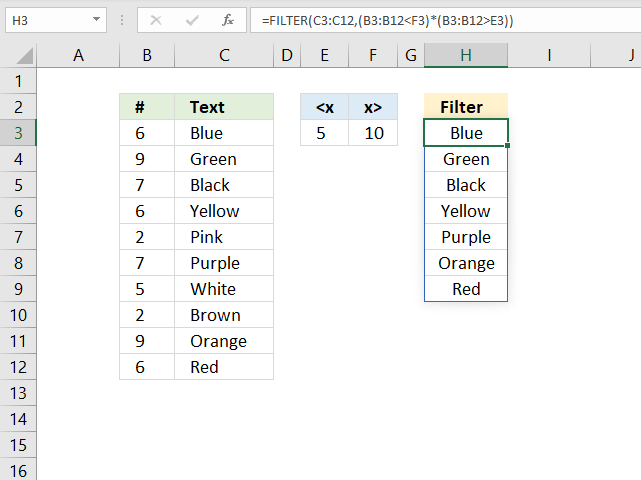Author: Oscar Cronquist Article last updated on May 17, 2021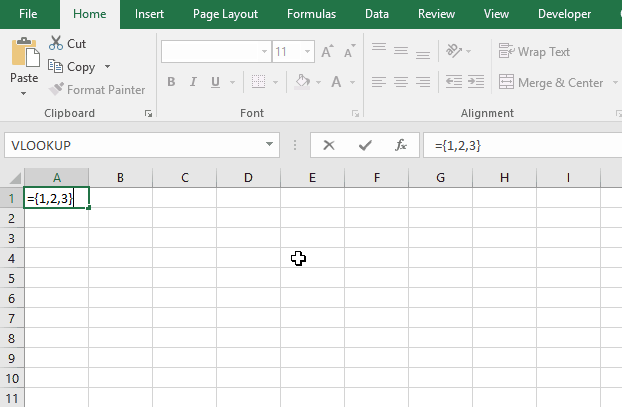This article explains the basics of array formulas, read this article if you never have used array formulas before. It opened up a new world to me and I am sure you will find many applications for these kinds of calculations.

## 1. Why are array formulas so powerful?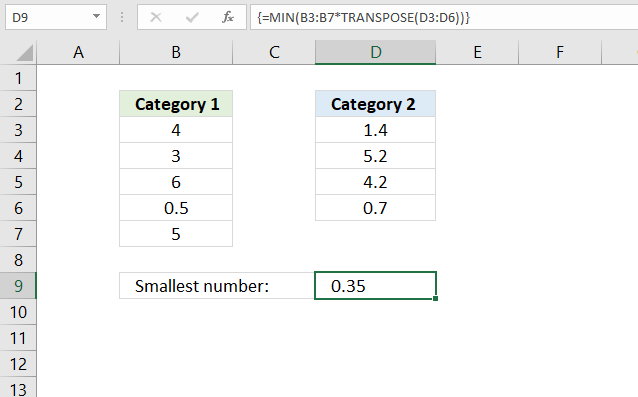They allow you to do things not possible with regular formulas, an array formula can do multiple calculations based on several values simultaneously. You can do some seriously complicated calculations, Excel is definitively a powerful tool.

The array formula example, demonstrated in the image above, performs calculations to multiple numbers simultaneously and returns the smallest number from those calculations.

It multiplies all numbers in column B with all numbers in column D and returns the smallest product of all numbers.

Array formula in cell D9:

=MIN(B3:B7*TRANSPOSE(D3:D6))

### Explaining formula in cell D9

#### Step 1 - Transpose numbers

It is not possible to multiply two vertical arrays containing a different amount of numbers. The TRANSPOSE function converts a vertical array to horizontal and vice versa.

However, if they were of identical size the result would not be what we are looking for anyway, the first number would be multiplied with the first value in the second array only. We want each value multipled with all numbers in the second array to calculate all combinations.

TRANSPOSE(D3:D6)

becomes

TRANSPOSE({1.4;5.2;4.2;0.7})

and returns {1.4,5.2,4.2,0.7}.

Note that the delimiting character is a semicolon in the vertical array and a comma in the horizontal array.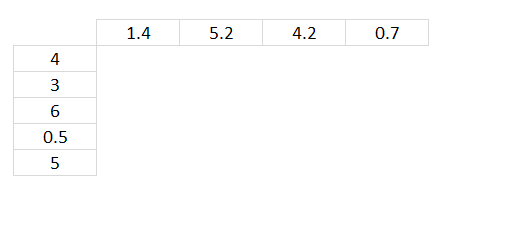The image above shows the numbers in the second array arranged horizontally.

#### Step 2 - Multiply numbers

B3:B7*TRANSPOSE(D3:D6)

becomes

B3:B7*{1.4,5.2,4.2,0.7}

becomes

{4;3;6;0.5;5}*{1.4,5.2,4.2,0.7}

and returns {5.6,20.8,16.8,2.8;4.2,15.6,12.6,2.1;8.4,31.2,25.2,4.2;0.7,2.6,2.1,0.35;7,26,21,3.5}.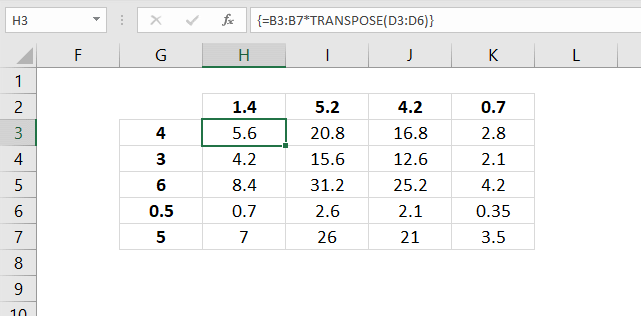The result is shown in cell range H3:K7 in the image above. Cell H3 shows the product of the numbers in cell H2 and G3, and so on.

#### Step 3 - Extract the smallest number in the array

The MIN function returns the smallest number from a cell range or an array.

MIN(B3:B7*TRANSPOSE(D3:D6))

becomes

MIN({5.6,20.8,16.8,2.8;4.2,15.6,12.6,2.1;8.4,31.2,25.2,4.2;0.7,2.6,2.1,0.35;7,26,21,3.5})

and returns 0.35 which is the smallest number in the array.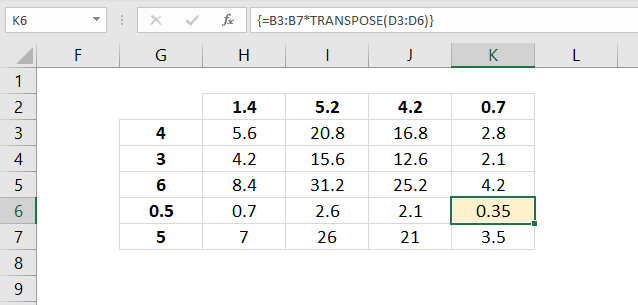The image below shows the result in cell D9, all these calculations were completed in one cell only.## 2. How to identify array formulas?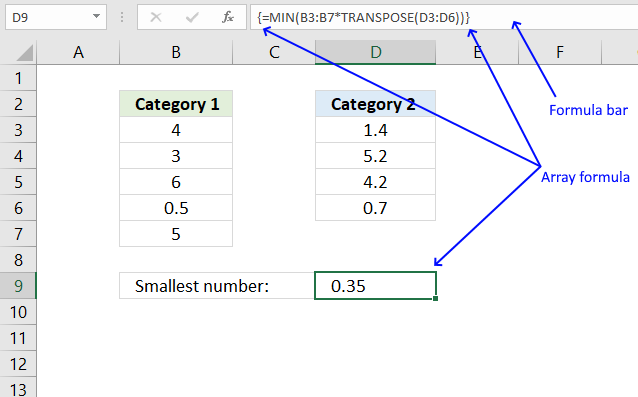The formula bar is above your worksheet, it may be empty or contain a value or a formula. The image above demonstrates an array formula in cell D9.

The formula bar shows what kind of formula the selected cell contains. An array formula begins with a curly bracket and ends with a curly bracket.

These curly brackets appear automatically if you enter the formula as an array formula, see next section below on how to do that.

## 3. How to enter an array formula?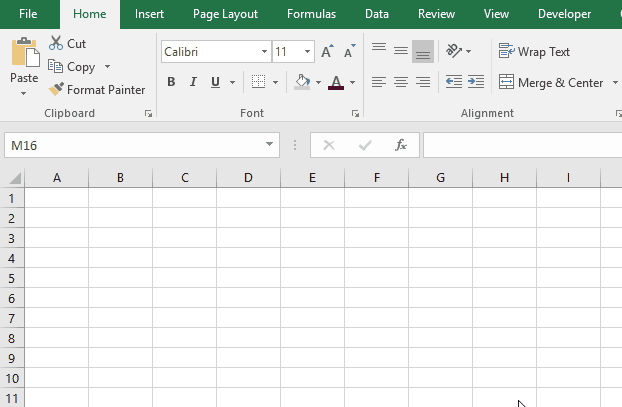Constructing an array in Excel is easy. Double press with left mouse button on cell A1 and type ={1,2,3} in the formula bar and hold and press CTRL + SHIFT simultaneously, then press Enter once. Release all keys.

The formula in the formula bar now shows a beginning and ending curly bracket. Don't enter those characters yourself. Make sure the formula bar looks like this: {={1,2,3}}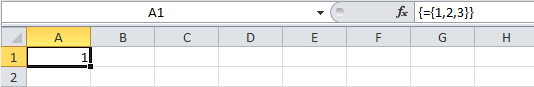If your formula bar looks like the picture above then you have made your first array! If not try again.

={1,2,3} is an array of constants separated by a delimiting character. This formula is entered in cell A1 but only one value in the array is displayed, why is that? The next section below explains why this happens.

## 4. Why is only one value shown? I have x values in the array?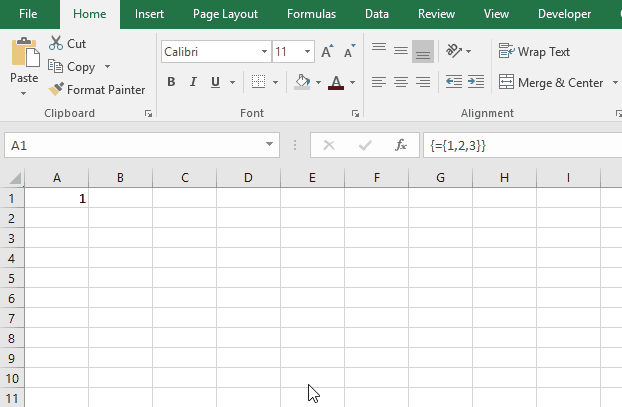There can only be one value in each cell. To be able to see all values enter the formula in cell range A1:C1

1. Select cell range A1:C1
2. Press with left mouse button on in the formula bar
3. Press and hold CTRL + SHIFT simultaneously
4. Press Enter
5. Release all keys

The animated picture above shows you how to extend an array formula from cell A1 to A1:C1, this allows you to see all values in the array.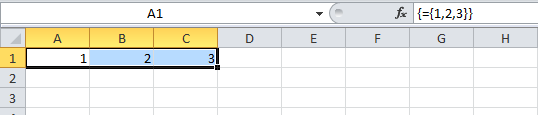You have now extended the formula to three cells and all values in the array are visible on the sheet, see picture above.

## 5. What are vertical and horizontal arrays?

You can construct arrays with a comma delimiter and a semicolon delimiter.

What is the difference? Use the comma when you want values horizontally and semicolon vertically. Look at the formula bar in this animated picture: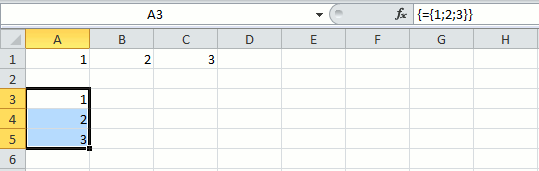You can also combine colons and semicolons. ={1,2;3,4} A comma tells excel that the next value is to the right of the first value. A semicolon puts the next value on the next row.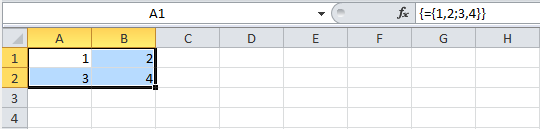I try to enter an array with text values but it doesn't work, why? Use double quotes around your values, like this: ={"A","B"}

## 6. How to use cell references in array formulas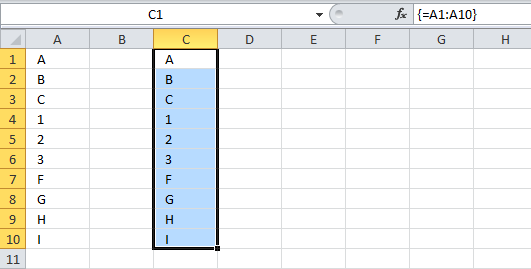All arrays presented above contain constants, meaning they don't change unless you edit them. This formula =A1:A10 contains a cell reference to A1:A10. The values in this formula change if you change a value in cell range A1:A10. Formula =A1:A10 does not contain any constants.

This means that you can use values on your sheet in your array formula. This is extremely useful.

## 7. How to quickly build an array

I want to construct an array from 1 to 1000? I don't want to enter all these values.
You don't have to, the ROW function can do that for you. =ROW(1:1000) creates an array from 1 to 1000.

## 8. How to hard code (literal) values in array formulas?

Can I hard code values from 1 to 1000 in a formula without entering them myself? Yes, you can. Type =ROW(1:1000) in the formula bar and press F9.

Excel converts =ROW(1:1000) to ={1,2,3, ... , 1000)

## 9. Is Ctrl + Shift + Enter always necessary?

Can I build an array formula without entering it as an array formula?
Yes, you often can. However, it makes the formula more complicated.
Read this post:  No more array formulas?

Excel 365 users enter array formulas as regular formulas, I recommend upgrading to Excel 365. There are so many new things in Excel 365.

## 10. Can I enter an array formula in merged cells?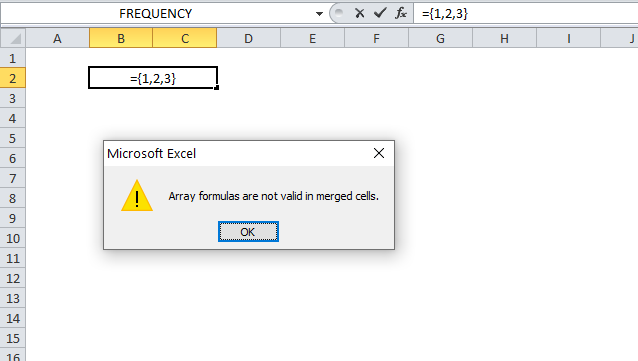Excel tells me "Array formulas are not valid in merged cells", how can I enter array formulas in merged cells?
Yes, you can enter array formulas in merged cells using a workaround: How to enter array formulas in merged cells however, I recommend that you avoid merged cells as much as possible.

## 11. Are there any exceptions?

Yes, both the SUMPRODUCT function and the LOOKUP function lets you build arrays without requiring you to enter them as an array formula.

How to use the SUMPRODUCT function

The SUMPRODUCT function calculates the product of corresponding values and then returns the sum of each multiplication.

How to use the LOOKUP function

Finds a value in a sorted cell range and returns a value on the same row.

The INDEX function lets you encapsulate an array so you don't need to enter it as an array formula:

Convert array formula to a regular formula

This article explains how to avoid array formulas if needed. It won't work with all array formulas, however, smaller ones […]

How to use the INDEX function

Gets a value in a specific cell range based on a row and column number.

## 12. Any caveats using array formulas?

A major disadvantage with array formulas is that if you double press with left mouse button on a cell containing an array formula and then press with left mouse button on another cell, the array formula changes to a regular formula. Beginner Excel users may not know about this and could possibly break your worksheet calculations.

However, this is not the case if you press the Escape key to exit editing an array formula or if you press CTRL + SHIFT + Enter.

Array formulas can slow down your worksheet calculations.

## 13. Alright, show me some useful array formulas?

5 easy ways to extract Unique Distinct Values

First, let me explain the difference between unique values and unique distinct values, it is important you know the difference […]

5 easy ways to VLOOKUP and return multiple values

This post explains how to lookup a value and return multiple values. No array formula required.

Repeat values across cells

This article explains how to repeat specific values based on a table, the table contains the items to be repeated […]

Cool, even more complicated calculations?

Count multiple text strings in a cell range

This article demonstrates an array formula that counts how many times multiple text strings exist in a cell range.  The […]

Dynamic scoreboard

This article demonstrates a scoreboard, displayed to the left, that sorts contestants based on total scores and refreshes instantly each […]

Find numbers closest to sum

Excelxor is such a great website for inspiration, I am really impressed by this post Which numbers add up to […]

Count overlapping days across multiple date ranges

This post demonstrates a formula in cell D16 that counts overlapping dates across multiple date ranges. The date ranges are […]